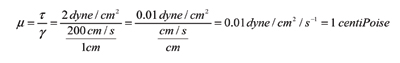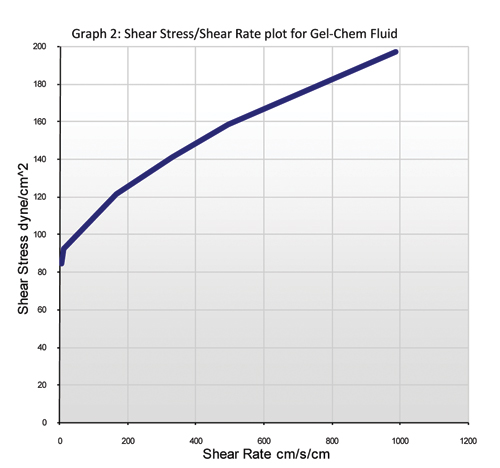# Why Is Drilling Fluid Non-Newtonian?Drilling fluid manuals generally differentiate between Newtonian and non-Newtonian fluids. This is an important distinction because the relationship between shear stress and shear rate, and thus the formulas for calculating viscosity, are different depending on whether the fluid is Newtonian or non-Newtonian.

The manuals classify drilling fluids as non-Newtonian and define non-Newtonian fluids as fluids that have a non-linear relationship between shear stress and shear rate. This definition of non-Newtonian fluids has limited value to the field technician because the definition describes the outcome of the graphical analysis technique rather than describing the physical reason why the fluid is non-Newtonian or why there is a non-linear relationship between shear stress and shear rate. In this article, we will explore what is meant by a linear and non-linear relationship and the physical reason for this difference.

## Linear RelationshipThe terms, linear and non-linear relationship are used in graphical analysis to describe the change in one variable given the change in the other variable when the values of each variable are plotted on a graph. Graph 1 shows the changes in the shear stress in a fluid at different shear rates. The shear stress (one of the variables) increases by 2 dyne/cm2 every time the shear rate increases by 200. From 0 cm/s/cm to 200 cm/s/cm, the shear stress goes up by 2 dyne/cm2; from 600 cm/s/cm to 800 cm/s/cm, the shear stress also increases by 2 dyne/cm2. Whenever the shear rate changes by 1 cm/s/cm, the shear stress changes by 0.1 dyne/cm2. This is true no matter how fast the fluid is moving within the velocities (shear rates) shown on the graph. The relationship between shear stress and shear rate is linear because for every incremental change in shear rate, there is the same incremental change in shear stress. If the fluid has a linear relationship, then the viscosity that is calculated using one pair of shear stress/shear rate values can be used to calculate the shear stress for any shear rate or the shear rate can be calculated for any shear stress. For example, the viscosity of the fluid at 200 cm/s/cm is:Using the viscosity from the above equation, we can calculate or predict what the shear stress would be at 800 cm/s/cm by simply multiplying 800 cm/s/cm by 0.01 dyne/cm2/s-1 to get 8 dyne/cm2.A linear relationship between shear stress and shear rate in a fluid makes it easy to calculate or predict what the shear stress will be at any shear rate, or vice-versa, once you know the relationship between the shear stress and the shear rate (i.e. how much one variable changes in relation to a change in the other variable).

A Newtonian fluid is defined as a fluid that has a linear relationship between shear stress and shear rate.

## Non-Linear Relationship

In a non-linear relationship, one variable does not change by the same amount for every incremental increase in the other variable. In Graph 2, the shear stress changed by 7.6 dyne/cm2 when the shear rate increased from 5 to 10 cm/s/cm, and from 500 to 1000 cm/s/cm, the shear rate increased by 38.3 dyne/cm2. From 5 to 10 cm/s/cm, the shear stress increased by 1.6 every time the shear rate increased by 1. From 500 to 1,000 cm/s/cm, the shear stress increase by 0.08 every time the shear rate increased by 1. At low shear rates (fluid velocities), an incremental increase in shear rate produces a high increase in shear stress, while at high shear rates, an incremental increase in shear rate produces only a small increase in shear stress.

The relationship between shear rate and shear stress is non-linear in this case because one variable (the shear stress) does not change by the same amount for every incremental change in the other variable (shear rate).The non-linear relationship between shear stress and shear rate makes it more complicated to calculate or make predictions about what shear stress will be at a certain shear rate based on what it was at some other shear rate. For example, the shear stress on Graph 2 at 165 cm/s/cm is 121.36 dyne/cm2. The viscosity using these two variable works out to be 0.74 dyne/cm2/s-1. If we try to calculate or predict what the shear stress will be at 500 cm/s/cm using the viscosity of 0.74 dyne/cm2/s-1, the calculation gives an answer of 370 dyne/cm2. Looking at the graph, we can see that the shear stress at 500 cm/s/cm is not even close to 370 dyne/cm2. This is because the shear stress does not change by the same amount for every incremental change in the shear rate and vice-versa.

A non-Newtonian fluid is defined as a fluid in which there is a non-linear relationship between shear stress and shear rate.

## Why Is There a Difference?

Graph 1 is a shear stress/shear rate plot for water and shows a linear relationship between the two variables, indicating a Newtonian fluid. Graph 2 is a shear stress/shear rate plot for a drill slurry made up of water and bentonite and shows a non-linear relationship between the two variables, indicating a non-Newtonian fluid. The only physical difference between the two fluids is the bentonite. A Newtonian fluid is a fluid that is made up entirely of a liquid, whereas a non-Newtonian fluid is a fluid that is made up of a liquid with a solid or “semi-solid” mixed into the liquid. The relationship between shear stress and shear rate is different at various shear rates in a non-Newtonian fluid because the interaction between the solids is different at different shear rates.

At low shear rates, the interaction between the solids is mostly due to electro-chemical forces of attraction. When we try to move the fluid, the fluid resists movement and produces a shear stress because of the electro-chemical bonds between the solids that are suspended in the fluid. To get the fluid moving, we have to break some of the electro-chemical bonds between the solids. The shear stress that we see in the low shear rate range is an indication of the strength of the electro-chemical bonds between the solids. In high quality gel-chem drilling fluids, the shear stress at low shear rates is high because the electro-chemical bonds between the solids is high. In a low quality drilling fluid, the shear stress at low shear rates is low because there is very little electro-chemical bonding between the solids.

At high shear rates, the interaction between the solids is dominated by friction between the solid particles as they move past one another. High shear stress increases at high shear rates is an indication of the amount of solids in the fluid. In high quality gel-chem fluids, the stress produced by friction is low, whereas in low quality fluids, the stress produced by friction is high.

## Conclusion

Drilling fluids are usually non-Newtonian because they are made up of a liquid and a solid or “semi-solid” combined. Newtonian fluids are all liquid, with no solids suspended in the liquid. In non-Newtonian fluids, there is a non-linear relationship between shear stress and shear rate because the interaction between the solids is different at different shear rates. When the fluid is not moving or moving very slow, the interaction between the solids is mostly the result of electro-chemical bonding. At high shear rates, the interaction between the solids is dominated by friction as the solids move past one another. High quality drilling fluids have high shear stress at low shear rates and low increases in shear stress at high shear rates.

If drilling fluids were Newtonian, an evaluation of the shear stress at one shear rate would be enough to evaluate the quality of the fluid and to calculate or predict the behavior of the fluid at different shear rates (velocities). But since drilling fluids are non-Newtonian, the quality of the drilling fluid is best assessed by measuring and evaluating the shear stress across a wide range of shear rates.

Manley Osbak is vice president engineering and estimating at The Crossing Co., Nisku, Alberta, Canada.